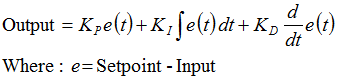Home » Flight control » PID controller

## PID controller

To the PID controller there is not much to say. See here.The SW implementation is also straight forward. Here you find a cool blog about it.

The implementation could look like this.

``````
void setPIDFactors()
{
kpr = 0.5; //PID constants for for roll angle
kir = 0.01;
kdr = 2.0;

kpp = 0.5; //PID constants for pitch angle
kip = 0.01;
kdp = 2.0;

kpy = 1.0; //PID constants for yaw angle
kiy = 0;
kdy = 0;

sampleFrequency = 333; //sample update rate of the MPU9250
}

void ComputePIDRoll()
{
errorR = 100 * (-setRoll - roll); //in this application the setRoll has to be negated; depends on the orientation matrix of the MPU9250
errSumR = errSumR + errorR / sampleFrequency;
if (errSumR * kir > 1000)
{
errSumRWatch = 1000;
}
else if (errSumR * kir < -1000)
{
errSumRWatch = -1000;
}
else errSumRWatch = errSumR;
dErrR = errorR - lastErrR;
outputR = outputR; //we build an average output over 5 samples;  holds the average value
outputR = outputR;
outputR = outputR;
outputR = outputR;
outputR = (kpr / 10.0 * errorR) + (kir * errSumR) + (kdr * dErrR);
outputR = (outputR + outputR + outputR + outputR + outputR) / 5; //
lastErrR = errorR;
}
``````

Pich and Yaw use a similar PID controller function.